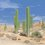# Polynomials sprint: How to solve a seemingly disgusting polynomial

On page 248, it is shown how to solve the polynomial $x^4+x^3+x^2+x+1=0$. In this note, I will explain how to solve the polynomial $x^8+x^7+x^6+x^5+x^4+x^3+x^2+x+1=0$ First we multiply both sides by $(x-1)$ to get $x^9-1=0\implies x^9=1$ Then since $x^9=1$, we can divide some terms by $x^9$ $x^8+x^7+x^6+x^5+x^4+x^3+x^2+x+1=$ $\dfrac{x^8}{x^9}+\dfrac{x^7}{x^9}+\dfrac{x^6}{x^9}+\dfrac{x^5}{x^9}+x^4+x^3+x^2+x+1=$ $\dfrac{1}{x}+\dfrac{1}{x^2}+\dfrac{1}{x^3}+\dfrac{1}{x^4}+x^4+x^3+x^2+x+1$ $\left(x+\dfrac{1}{x}\right)+\left(x^2+\dfrac{1}{x^2}\right)+\left(x^3+\dfrac{1}{x^3}\right)+\left(x^4+\dfrac{1}{x^4}\right)+1=0$ Now we let $y=x+\dfrac{1}{x}$ so we have $(y)+(y^2-2)+(y^3-3y)+((y^2-2)^2-2)+1=0$ which after simplification becomes $y^4+y^3-3y^2-2y+1=0$ Using the Rational Root Theorem (discussed on page 246) we quickly find that $y=-1$ is a root and factor the polynomial as $(y+1)(y^3-3y+1)$ Now the rest is simple! We can use the cubic solving method discussed on pages 247-248 to find the roots of $y^3-3y+1$ Then simple quadratic bashing gives us our roots!Note by Nathan Ramesh
6 years, 12 months ago

This discussion board is a place to discuss our Daily Challenges and the math and science related to those challenges. Explanations are more than just a solution — they should explain the steps and thinking strategies that you used to obtain the solution. Comments should further the discussion of math and science.

When posting on Brilliant:

• Use the emojis to react to an explanation, whether you're congratulating a job well done , or just really confused .
• Ask specific questions about the challenge or the steps in somebody's explanation. Well-posed questions can add a lot to the discussion, but posting "I don't understand!" doesn't help anyone.
• Try to contribute something new to the discussion, whether it is an extension, generalization or other idea related to the challenge.
• Stay on topic — we're all here to learn more about math and science, not to hear about your favorite get-rich-quick scheme or current world events.

MarkdownAppears as
*italics* or _italics_ italics
**bold** or __bold__ bold
- bulleted- list
• bulleted
• list
1. numbered2. list
1. numbered
2. list
Note: you must add a full line of space before and after lists for them to show up correctly
paragraph 1paragraph 2

paragraph 1

paragraph 2

[example link](https://brilliant.org)example link
> This is a quote
This is a quote
    # I indented these lines
# 4 spaces, and now they show
# up as a code block.

print "hello world"
# I indented these lines
# 4 spaces, and now they show
# up as a code block.

print "hello world"
MathAppears as
Remember to wrap math in $$ ... $$ or $ ... $ to ensure proper formatting.
2 \times 3 $2 \times 3$
2^{34} $2^{34}$
a_{i-1} $a_{i-1}$
\frac{2}{3} $\frac{2}{3}$
\sqrt{2} $\sqrt{2}$
\sum_{i=1}^3 $\sum_{i=1}^3$
\sin \theta $\sin \theta$
\boxed{123} $\boxed{123}$

## Comments

Sort by:

Top Newest

Or, we can use the 9th roots of unity.

- 6 years, 12 months ago

Log in to reply

Yes, what happened to the classic roots of unity? Roots are just $e^{2ki\pi/9}$with $k=1\to 8$.

- 6 years, 12 months ago

Log in to reply

Except all roots of unity give you is $e^{\frac{2\pi i}{9}}$ and not a numerical answer with radicals. The method here allows you to compute $\sin {40^{\circ}}$

- 6 years, 12 months ago

Log in to reply

Or you can also just do some Euler's formula with $e^{\dfrac{2\pi i}{9}}$.

- 6 years, 12 months ago

Log in to reply

ummmm which euler's formula?

- 6 years, 12 months ago

Log in to reply

Where i can find this book

- 6 years, 5 months ago

Log in to reply

$e^{i\phi}$ = cos$\phi$ + ${i}$sin$\phi$ ... substituting $\pi$ gives $e^{i\pi}$ = -1, where ${i}$ = $\sqrt{-1}$

- 6 years, 5 months ago

Log in to reply

$e^{i\theta}=\text{cis}(\theta)$

- 6 years, 12 months ago

Log in to reply

How does that let you solve for $\sin{40^{\circ}}$

- 6 years, 12 months ago

Log in to reply

wow

- 6 years, 12 months ago

Log in to reply

:o

- 6 years, 12 months ago

Log in to reply

2 Daniels

- 6 years, 12 months ago

Log in to reply

2 Lius

- 6 years, 12 months ago

Log in to reply

2 14-year-old-Daniel-Lius. Enough to get a conversation off-topic.

- 6 years, 12 months ago

Log in to reply

2 14-year-old-Daniel-Lius-residing-in-USA. conversation closed.

- 6 years, 12 months ago

Log in to reply

Oh God, this is not good. One Daniel is enough for me, but two? Double Trouble.

- 6 years, 12 months ago

Log in to reply

I reshared your note. :D

- 6 years, 12 months ago

Log in to reply

@Nathan Ramesh Can you add this to the Roots of Unity Applications Wiki page?

Select "Write a summary", and then copy-paste your text into it (with minor formatting adjustments if relevant. Thanks!

Staff - 6 years, 8 months ago

Log in to reply

Done! I put it at the bottom. Let me know if it is bugged (I posted it from my phone). Thanks!

- 6 years, 8 months ago

Log in to reply

Yes I used the same to expand cosnx

- 6 years, 5 months ago

Log in to reply

Where are all other pages?

- 6 years, 5 months ago

Log in to reply

×

Problem Loading...

Note Loading...

Set Loading...